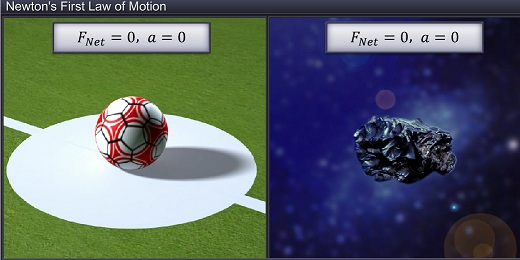Notes On Newton's First Law of Motion - CBSE Class 11 Physics
Newton’s Laws of Motion have their roots in Galileo’s Law of Inertia. Newton’s First Law of Motion is almost the same as Galileo’s Law of Inertia. Newton’s First Law of Motion states that: “Every object continues to be in its state of rest or of uniform motion in a straight line unless compelled by some external force to act otherwise.”   When a body is at rest, it has no acceleration. Similarly, a body in uniform motion in a straight line does not accelerate.Based on these facts, we can express Newton’s First Law of Motion in two more very useful forms. One: if the net external force on a body is zero, its acceleration is zero and vice-versa. Two: “A body accelerates only when a net external force acts on it.” These two forms of the law are extensively used while solving problems in kinetics.

#### Summary

Newton’s Laws of Motion have their roots in Galileo’s Law of Inertia. Newton’s First Law of Motion is almost the same as Galileo’s Law of Inertia. Newton’s First Law of Motion states that: “Every object continues to be in its state of rest or of uniform motion in a straight line unless compelled by some external force to act otherwise.”   When a body is at rest, it has no acceleration. Similarly, a body in uniform motion in a straight line does not accelerate.Based on these facts, we can express Newton’s First Law of Motion in two more very useful forms. One: if the net external force on a body is zero, its acceleration is zero and vice-versa. Two: “A body accelerates only when a net external force acts on it.” These two forms of the law are extensively used while solving problems in kinetics.

Previous
Next